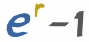# Compound Interest: Periodic Compounding

You
can skip straight down to Periodic Compounding.

## Quick Explanation of Compound Interest

With Compound Interest, you work out the interest for the first period, add it to the total, and then calculate the interest for the next period, and so on ..., like this:But adding 10% interest is the same as multiplying by 1.10 (explained here)

So it also works like this:In fact we can go from the Start to Year 5 if we multiply 5 times using Exponents (or Powers):## The Formula

This is the formula for Compound Interest (like above but using letters instead of numbers):### Example: \$1,000 invested at 10% for 5 Years:

Present Value PV = \$1,000

Interest Rate is 10%, which as a decimal r = 0.10

Number of Periods n = 5

PV × (1 + r)n = FV
\$1,000 × (1 + 0.10)5 = FV
\$1,000 × 1.105 = \$1,610.51

Now we can choose different values, such as an interest rate of 6%:

### Example: \$1,000 invested at 6% for 5 Years:

Present Value PV = \$1,000

Interest Rate is 6%, which as a decimal r = 0.06

Number of Periods n = 5

PV × (1 + r)n = FV
\$1,000 × (1 + 0.06)5 = FV
\$1,000 × 1.065 = \$1,338.23

## Periodic Compounding (Within The Year)

But sometimes interest is charged Yearly ...

... but it is calculated more than once within the year, with the interest added each time ...

... so there are compoundings within the Year.

### Example: "10%, Compounded Semiannually"

Semiannual means twice a year. So the 10% is split into two:

• 5% halfway through the year,
• and another 5% at the end of the year,

but each time it is compounded (meaning the interest is added to the total):10%, Compounded Semiannually

This results in \$1,102.50, which is equal to 10.25%, not 10%

### Two Annual Interest Rates?

Yes, there are two annual interest rates:

 Example 10% The Nominal Rate (the rate they mention) 10.25% The Effective Annual Rate (the rate after compounding)

The Effective Annual Rate is what actually gets paid!

When interest is compounded within the year, the Effective Annual Rate is higher than the rate mentioned.

How much higher depends on the interest rate, and how many times it is compounded within the year.

### Working It Out

Let's come up with a formula to work out the Effective Annual Rate if we know:

• the rate mentioned (the Nominal Rate, "r")
• how many times it is compounded ("n")

Our task is to take an interest rate (like 10%) and chop it up into "n" periods, compounding each time.

From the Compound Interest formula (shown above) we can compound "n" periods using

FV = PV (1+r)n

But the interest rate won't be "r", because it has to be chopped into "n" periods like this:

r / n

So we change the compounding formula into:

This is the formula for Periodic Compounding:

FV = PV (1+(r/n))n

where FV = Future Value
PV = Present Value
r = annual interest rate
n = number of periods within the year

Let's try it on our "10%, Compounded Semiannually" example:

FV = \$1,000 (1+(0.10/2))2 = \$1,000(1.05)2 = \$1,000 × 1.1025 = \$1,102.50

That worked! But we want to know what the new interest rate is, we don't want the dollar values in there, so let's remove them:

(1+(r/n))n = (1.05)2 = 1.1025

That has the interest rate in there (0.1025 = 10.25%), but we should subtract the extra 1:

(1+(r/n))n − 1 = 0.1025 = 10.25%

And so the formula is:

Effective Annual Rate = (1+(r/n))n − 1

### Example: what rate do you get when the ad says "6% compounded monthly"?

r = 0.06 (which is 6% as a decimal)
n = 12

Effective Annual Rate = (1+(r/n))n − 1

= (1+(0.06/12))12 − 1

= (1.005)12 − 1 = 0.06168 = 6.168%

So you actually get 6.168%

### Example: 7% interest, compounded 4 times a year.

r = 0.07 (which is 7% as a decimal)
n = 4

So:

FV = PV (1+(0.07/4))4

FV = PV (1+(0.07/4))4

FV = PV (1.0719...)

The effective annual rate is 7.19%

So remember:

r / n Chop the interest rate into "n" periods Compound that "n" times: Don't forget to subtract the "1"

## Table of Values

Here are some example values. Notice that compounding has a very small effect when the interest rate is small, but a large effect for high interest rates.

Compounding Periods   1.00% 5.00% 10.00% 20.00% 100.00%
Yearly 1   1.00% 5.00% 10.00% 20.00% 100.00%
Semiannually 2   1.00% 5.06% 10.25% 21.00% 125.00%
Quarterly 4   1.00% 5.09% 10.38% 21.55% 144.14%
Monthly 12   1.00% 5.12% 10.47% 21.94% 161.30%
Daily 365   1.01% 5.13% 10.52% 22.13% 171.46%
... ...
Continuously Infinite   1.01% 5.13% 10.52% 22.14% 171.83%

### Continuously?

Yes, if you have smaller and smaller periods (hourly, minutely, etc) you eventually reach a limit, and we even have a formula for it:Continuous Compounding Formula
Note: e=2.71828..., which is Euler's number.

### Example:Continuous Compounding for 20%

e0.20 − 1 = 1.2214... − 1 = 0.2214...

## Using It

Now that you can calculate the Effective Annual Rate (for specific periods, or continuous), we can use it in any normal compound interest calculations.

### Example: Continuous Compounding of \$10,000 for 2 years at 8%

Continuous Compounding for 8% is: e0.08 − 1 = 1.08329... − 1 = 0.08329...

Over 2 years (see Compound Interest) we get:

FV = PV × (1+r)n

FV = \$10,000 × (1+0.08329)2

FV = \$10,000 × 1.173511... = \$11,735.11

## Summary

Effective Annual Rate = (1+(r/n))n − 1

Where:

• r = Nominal Rate (the rate they mention)
• n = number of periods that are compounded (example: monthly=12)